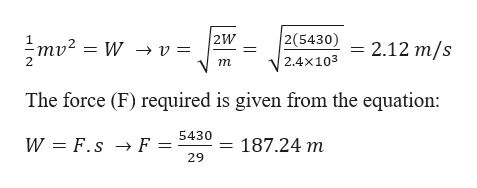# A mechanic pushes a 2.40 ✕ 103-kg car from rest to a speed of v, doing 5,430 J of work in the process. During this time, the car moves 29.0 m. Neglecting friction between car and road, find v and the horizontal force exerted on the car.(a) the speed v  m/s (b) the horizontal force exerted on the car (Enter the magnitude.)

Question
9 views

A mechanic pushes a 2.40 ✕ 103-kg car from rest to a speed of v, doing 5,430 J of work in the process. During this time, the car moves 29.0 m. Neglecting friction between car and road, find v and the horizontal force exerted on the car.

(a) the speed v
m/s

(b) the horizontal force exerted on the car (Enter the magnitude.)
check_circle

Step 1

Given information:

Mass of the car (m) = 2.4 × 103 kg

The work done in moving the car (W) = 5430 J

The distance through which the car is moved (s) = 29.0 m

Step 2

The velocity (v) of the car after the work is done is given by equating ...help_outlineImage Transcriptionclose2(5430) mv? = W → v= = 2.12 m/s 2.4x103 т The force (F) required is given from the equation: 5430 W = F.s → F = 187.24 m 29 fullscreen

### Want to see the full answer?

See Solution

#### Want to see this answer and more?

Solutions are written by subject experts who are available 24/7. Questions are typically answered within 1 hour.*

See Solution
*Response times may vary by subject and question.
Tagged in

### Work,Power and Energy